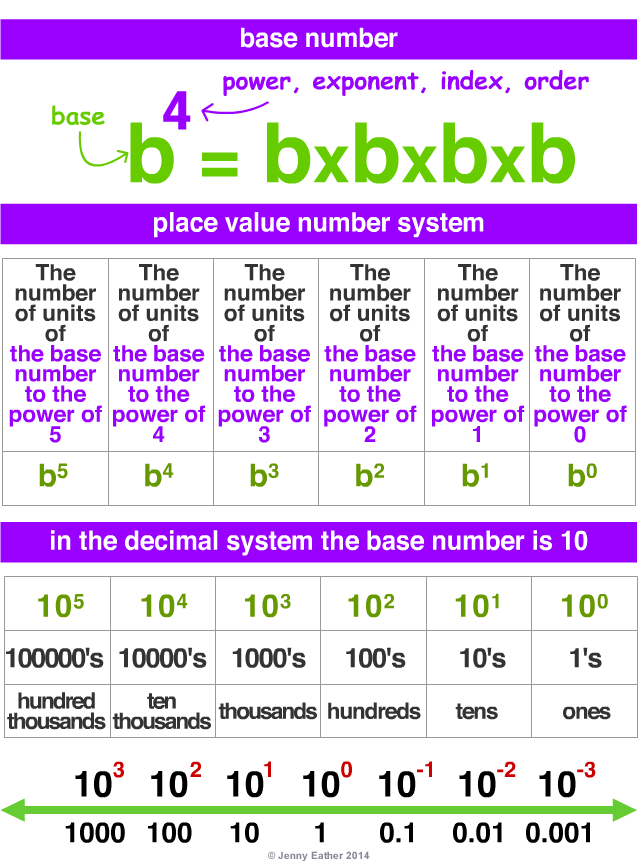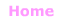Aa Bb Cc Dd Ee Ff Gg Hh Ii Jj Kk Ll Mm Nn Oo Pp Qq Rr Ss Tt Uu Vv Ww Xx Yy Zz

Bb

base number

• a number to be raised to a power, exponent, index or order.
These words all mean the same thing ...
• a small number placed
to the upper-right of the base number which shows
how many copies of the base number
are multiplied together.

EXAMPLES:
101 = 10
102 = 10 x 10
103 = 10 x 10 x 10
104 = 10 x 10 x 10 x 10
105 = 10 x 10 x 10 x 10 x 10
...

base numbers and number systems

• a base number is the basis of a place value number system.
• successive powers of the base number
are used for each column.
• the decimal system uses 10 as its base number
so it is called a Base-10 system.
• the binary system uses 2 as its base number so
it is called a Base-2 system.

EXAMPLE:© Jenny Eather 2014. All rights reserved.